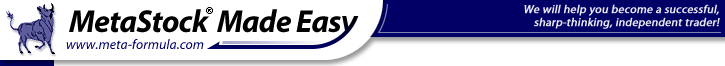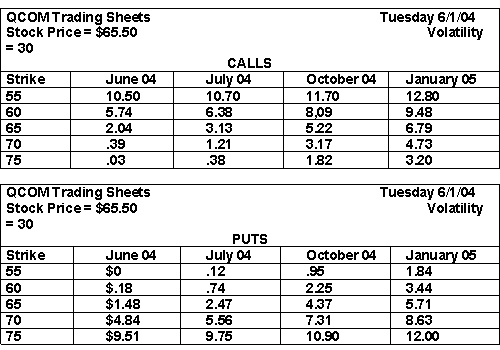Provided By Options University

We have demonstrated that vertical option spreads have intrinsic value, and that we can roughly determine their value by comparing stock price to strike prices. There is another relationship that can help investors determine value. That is the relationship that exists between corresponding vertical option spreads.

When we use the term corresponding we mean the same month, the same strikes in the same stock. The only difference is between calls and puts. For example, the XYZ Sept. 30  35 vertical call spreads corresponding option spreads would be the XYZ Sept. 30  35 vertical put spread. Similarly, the ABC June 70  80 put spreads corresponding option spreads would be the ABC June 70 80 call spread.

The importance of understanding the relationship of corresponding vertical option spreads is that the sum of a vertical call spread and its corresponding vertical put spread is going to be equal to the difference between the two strikes.

If the April 30  35 call option spreads trades at \$2.00, then the April 30  35 put spread will be worth \$3.00. Lets review this. The difference of the two strikes is \$5.00 and the cost of the call spread is \$2.00. That means the cost of the put option spreads will be \$3.00. The chart below is a floor traders pricing sheet that shows where individual options are trading and what they are worth based on each traders individual inputs.

From this we can calculate the price of any of our option spreads. Pick any vertical spread. Now, calculate the value of a vertical call spread or a vertical put spread. Once youve done that, calculate the value of its corresponding vertical spread. Add the two option spreads together and see if that sum is equal to the difference between the two strikes. Perform the calculations several times on different vertical spreads. Try it on \$5, \$10 and even \$15 spreads.Inside you’ll learn...

• How to design a winning system from scratch and exactly what to do to supercharge your current stock trading system!

• The one ingredient you literally "Drop" into your stock trading system that can triple your profit!

• How to use “secret” money management techniques to minimize your risk.

• The tools the professionals use and how you can get huge discounts (charting software, data, etc).

• And you'll also get a FREE copy of David Jenyns’ complete Ultimate Trading Systems Course…

 Name : E-Mail :